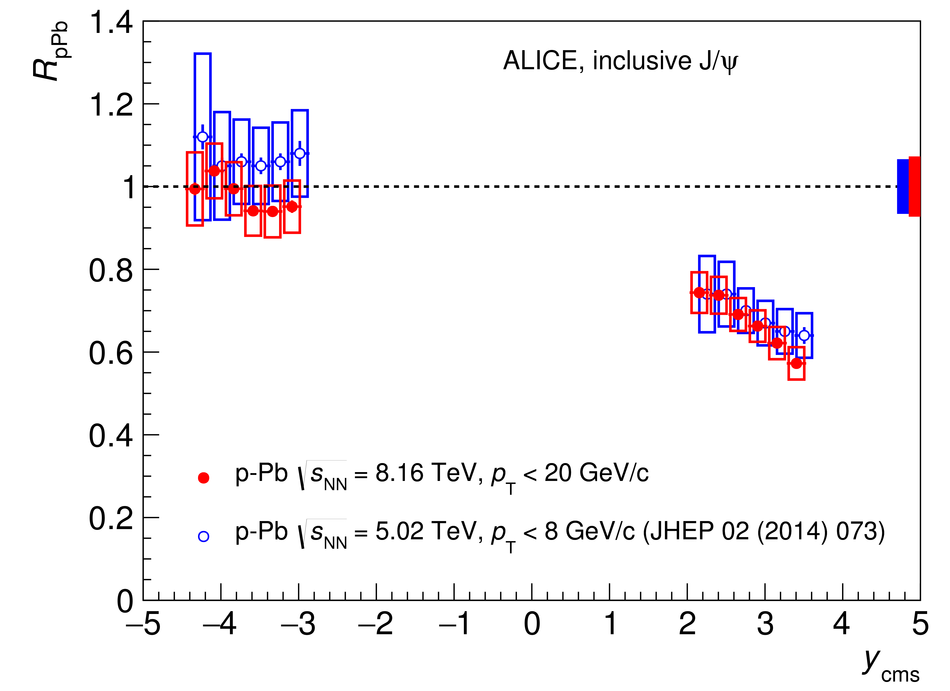# Figure 4

 The inclusive J/$\psi$ nuclear modification factor in \mbox{p--Pb} and \mbox{Pb--p} collisions at $\sqrt{s_{\rm NN}}=8.16$ TeV, as a function of $y_{\rm {cms}}$. The horizontal bars correspond to the bin size. The vertical error bars represent the statistical uncertainties, the boxes around the points the uncorrelated systematic uncertainties. Correlated uncertainties are shown as a filled box around unity for each energy. The results are compared with those for \mbox{p--Pb} and \mbox{Pb--p} collisions at $\sqrt{s_{\rm NN}}=5.02$ TeV~. The latter have been plotted at slightly shifted $y_{\rm cms}$ values, for better visibility.